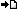RegisterMember LoginForgot Password ?? PHP , ASP , ASP.NET, VB.NET, C#, Java , jQuery , Android , iOS , Windows Phone

## Registered : 109,016

 HOME > PHP Manual > Arrays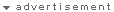## ลองใช้ค้นหาข้อมูล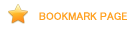## Arrays

An array in PHP is actually an ordered map. A map is a type that associates values to keys. This type is optimized for several different uses; it can be treated as an array, list (vector), hash table (an implementation of a map), dictionary, collection, stack, queue, and probably more. As array values can be other arrays, trees and multidimensional arrays are also possible.

Explanation of those data structures is beyond the scope of this manual, but at least one example is provided for each of them. For more information, look towards the considerable literature that exists about this broad topic.

### Syntax

#### Specifying with array()

An array can be created by the array() language construct. It takes as parameters any number of comma-separated key => value pairs.

```array(  key =>  value
, ...
)
// key may only be an integer or string
// value may be any value of any type```
``` <?php\$arr = array("foo" => "bar", 12 => true);echo \$arr["foo"]; // barecho \$arr;    // 1?> ```

A key may be either an integer or a string. If a key is the standard representation of an integer, it will be interpreted as such (i.e. "8" will be interpreted as 8, while "08" will be interpreted as "08"). Floats in key are truncated to integer. The indexed and associative array types are the same type in PHP, which can both contain integer and string indices.

A value can be any PHP type.

``` <?php\$arr = array("somearray" => array(6 => 5, 13 => 9, "a" => 42));echo \$arr["somearray"];    // 5echo \$arr["somearray"];   // 9echo \$arr["somearray"]["a"];  // 42?> ```

If a key is not specified for a value, the maximum of the integer indices is taken and the new key will be that value plus 1. If a key that already has an assigned value is specified, that value will be overwritten.

``` <?php// This array is the same as ...array(5 => 43, 32, 56, "b" => 12);// ...this arrayarray(5 => 43, 6 => 32, 7 => 56, "b" => 12);?> ```
Warning

Before PHP 4.3.0, appending to an array in which the current maximum key was negative would create a new key as described above. Since PHP 4.3.0, the new key will be 0.

Using TRUE as key will evaluate to integer 1 as a key. Using FALSE as key will evaluate to integer 0 as a key. Using NULL as a key will evaluate to the empty string. Using the empty string as a key will create (or overwrite) a key with the empty string and its value; it is not the same as using empty brackets.

Arrays and objects can not be used as keys. Doing so will result in a warning: Illegal offset type.

#### Creating/modifying with square bracket syntax

An existing array can be modified by explicitly setting values in it.

This is done by assigning values to the array, specifying the key in brackets. The key can also be omitted, resulting in an empty pair of brackets ([]).

```\$arr[key] = value;
\$arr[] = value;
// key may be an integer or string
// value may be any value of any type```

If \$arr doesn't exist yet, it will be created, so this is also an alternative way to create an array. To change a certain value, assign a new value to that element using its key. To remove a key/value pair, call the unset() function on it.

``` <?php\$arr = array(5 => 1, 12 => 2);\$arr[] = 56;    // This is the same as \$arr = 56;                // at this point of the script\$arr["x"] = 42; // This adds a new element to                // the array with key "x"                unset(\$arr); // This removes the element from the arrayunset(\$arr);    // This deletes the whole array?> ```

Note: As mentioned above, if no key is specified, the maximum of the existing integer indices is taken, and the new key will be that maximum value plus 1. If no integer indices exist yet, the key will be 0 (zero). If a key that already has a value is specified, that value will be overwritten.
Note that the maximum integer key used for this need not currently exist in the array. It need only have existed in the array at some time since the last time the array was re-indexed. The following example illustrates:

``` <?php// Create a simple array.\$array = array(1, 2, 3, 4, 5);print_r(\$array);// Now delete every item, but leave the array itself intact:foreach (\$array as \$i => \$value) {    unset(\$array[\$i]);}print_r(\$array);// Append an item (note that the new key is 5, instead of 0).\$array[] = 6;print_r(\$array);// Re-index:\$array = array_values(\$array);\$array[] = 7;print_r(\$array);?> ```

The above example will output:

```Array
(
 => 1
 => 2
 => 3
 => 4
 => 5
)
Array
(
)
Array
(
 => 6
)
Array
(
 => 6
 => 7
)

```

### Useful functions

There are quite a few useful functions for working with arrays. See the array functions section.

Note: The unset() function allows removing keys from an array. Be aware that the array will not be reindexed. If a true "remove and shift" behavior is desired, the array can be reindexed using the array_values() function.

``` <?php\$a = array(1 => 'one', 2 => 'two', 3 => 'three');unset(\$a);/* will produce an array that would have been defined as   \$a = array(1 => 'one', 3 => 'three');   and NOT   \$a = array(1 => 'one', 2 =>'three');*/\$b = array_values(\$a);// Now \$b is array(0 => 'one', 1 =>'three')?> ```

The foreach control structure exists specifically for arrays. It provides an easy way to traverse an array.

### Array do's and don'ts

#### Why is \$foo[bar] wrong?

Always use quotes around a string literal array index. For example, \$foo['bar'] is correct, while \$foo[bar] is not. But why? It is common to encounter this kind of syntax in old scripts:

``` <?php\$foo[bar] = 'enemy';echo \$foo[bar];// etc?> ```

This is wrong, but it works. The reason is that this code has an undefined constant (bar) rather than a string ('bar' - notice the quotes). PHP may in future define constants which, unfortunately for such code, have the same name. It works because PHP automatically converts a bare string (an unquoted string which does not correspond to any known symbol) into a string which contains the bare string. For instance, if there is no defined constant named bar, then PHP will substitute in the string 'bar' and use that.

Note: This does not mean to always quote the key. Do not quote keys which are constants or variables, as this will prevent PHP from interpreting them.

``` <?phperror_reporting(E_ALL);ini_set('display_errors', true);ini_set('html_errors', false);// Simple array:\$array = array(1, 2);\$count = count(\$array);for (\$i = 0; \$i < \$count; \$i++) {    echo "\nChecking \$i: \n";    echo "Bad: " . \$array['\$i'] . "\n";    echo "Good: " . \$array[\$i] . "\n";    echo "Bad: {\$array['\$i']}\n";    echo "Good: {\$array[\$i]}\n";}?> ```
The above example will output:
```Checking 0:
Notice: Undefined index:  \$i in /path/to/script.html on line 9
Good: 1
Notice: Undefined index:  \$i in /path/to/script.html on line 11
Good: 1

Checking 1:
Notice: Undefined index:  \$i in /path/to/script.html on line 9
Good: 2
Notice: Undefined index:  \$i in /path/to/script.html on line 11
Good: 2

```

More examples to demonstrate this behaviour:

``` <?php// Show all errorserror_reporting(E_ALL);\$arr = array('fruit' => 'apple', 'veggie' => 'carrot');// Correctprint \$arr['fruit'];  // appleprint \$arr['veggie']; // carrot// Incorrect.  This works but also throws a PHP error of level E_NOTICE because// of an undefined constant named fruit// // Notice: Use of undefined constant fruit - assumed 'fruit' in...print \$arr[fruit];    // apple// This defines a constant to demonstrate what's going on.  The value 'veggie'// is assigned to a constant named fruit.define('fruit', 'veggie');// Notice the difference nowprint \$arr['fruit'];  // appleprint \$arr[fruit];    // carrot// The following is okay, as it's inside a string. Constants are not looked for// within strings, so no E_NOTICE occurs hereprint "Hello \$arr[fruit]";      // Hello apple// With one exception: braces surrounding arrays within strings allows constants// to be interpretedprint "Hello {\$arr[fruit]}";    // Hello carrotprint "Hello {\$arr['fruit']}";  // Hello apple// This will not work, and will result in a parse error, such as:// Parse error: parse error, expecting T_STRING' or T_VARIABLE' or T_NUM_STRING'// This of course applies to using superglobals in strings as wellprint "Hello \$arr['fruit']";print "Hello \$_GET['foo']";// Concatenation is another optionprint "Hello " . \$arr['fruit']; // Hello apple?> ```

When error_reporting is set to show E_NOTICE level errors (by setting it to E_ALL, for example), such uses will become immediately visible. By default, error_reporting is set not to show notices.

As stated in the syntax section, what's inside the square brackets ('[' and ']') must be an expression. This means that code like this works:

``` <?phpecho \$arr[somefunc(\$bar)];?> ```

This is an example of using a function return value as the array index. PHP also knows about constants:

``` <?php\$error_descriptions[E_ERROR]   = "A fatal error has occured";\$error_descriptions[E_WARNING] = "PHP issued a warning";\$error_descriptions[E_NOTICE]  = "This is just an informal notice";?> ```

Note that E_ERROR is also a valid identifier, just like bar in the first example. But the last example is in fact the same as writing:

``` <?php\$error_descriptions = "A fatal error has occured";\$error_descriptions = "PHP issued a warning";\$error_descriptions = "This is just an informal notice";?> ```

because E_ERROR equals 1, etc.

##### So why is it bad then?

At some point in the future, the PHP team might want to add another constant or keyword, or a constant in other code may interfere. For example, it is already wrong to use the words empty and default this way, since they are reserved keywords.

Note: To reiterate, inside a double-quoted string, it's valid to not surround array indexes with quotes so "\$foo[bar]" is valid. See the above examples for details on why as well as the section on variable parsing in strings.

### Converting to array

For any of the types: integer, float, string, boolean and resource, converting a value to an array results in an array with a single element with index zero and the value of the scalar which was converted. In other words, (array)\$scalarValue is exactly the same as array(\$scalarValue).

If an object is converted to an array, the result is an array whose elements are the object's properties. The keys are the member variable names, with a few notable exceptions: private variables have the class name prepended to the variable name; protected variables have a '*' prepended to the variable name. These prepended values have null bytes on either side. This can result in some unexpected behaviour:

``` <?phpclass A {    private \$A; // This will become '\0A\0A'}class B extends A {    private \$A; // This will become '\0B\0A'    public \$AA; // This will become 'AA'}var_dump((array) new B());?> ```

The above will appear to have two keys named 'AA', although one of them is actually named '\0A\0A'.

Converting NULL to an array results in an empty array.

### Comparing

It is possible to compare arrays with the array_diff() function and with array operators.

### Examples

The array type in PHP is very versatile. Here are some examples:

``` <?php// this\$a = array( 'color' => 'red',            'taste' => 'sweet',            'shape' => 'round',            'name'  => 'apple',                       4        // key will be 0          );// is completely equivalent with\$a['color'] = 'red';\$a['taste'] = 'sweet';\$a['shape'] = 'round';\$a['name']  = 'apple';\$a[]        = 4;        // key will be 0\$b[] = 'a';\$b[] = 'b';\$b[] = 'c';// will result in the array array(0 => 'a' , 1 => 'b' , 2 => 'c'),// or simply array('a', 'b', 'c')?> ```

Example #1 Using array()

``` <?php// Array as (property-)map\$map = array( 'version'    => 4,              'OS'         => 'Linux',              'lang'       => 'english',              'short_tags' => true            );            // strictly numerical keys\$array = array( 7,                8,                0,                156,                -10              );// this is the same as array(0 => 7, 1 => 8, ...)\$switching = array(         10, // key = 0                    5    =>  6,                    3    =>  7,                     'a'  =>  4,                            11, // key = 6 (maximum of integer-indices was 5)                    '8'  =>  2, // key = 8 (integer!)                    '02' => 77, // key = '02'                    0    => 12  // the value 10 will be overwritten by 12                  );                  // empty array\$empty = array();         ?> ```

Example #2 Collection

``` <?php\$colors = array('red', 'blue', 'green', 'yellow');foreach (\$colors as \$color) {    echo "Do you like \$color?\n";}?> ```

The above example will output:

```Do you like red?
Do you like blue?
Do you like green?
Do you like yellow?

```

Changing the values of the array directly is possible since PHP 5 by passing them by reference. Before that, a workaround is necessary:

Example #3 Collection

``` <?php// PHP 5foreach (\$colors as &\$color) {    \$color = strtoupper(\$color);}unset(\$color); /* ensure that following writes to\$color will not modify the last array element */// Workaround for older versionsforeach (\$colors as \$key => \$color) {    \$colors[\$key] = strtoupper(\$color);}print_r(\$colors);?> ```

The above example will output:

```Array
(
 => RED
 => BLUE
 => GREEN
 => YELLOW
)

```

This example creates a one-based array.

Example #4 One-based index

``` <?php\$firstquarter  = array(1 => 'January', 'February', 'March');print_r(\$firstquarter);?> ```

The above example will output:

```Array
(
 => 'January'
 => 'February'
 => 'March'
)

```

Example #5 Filling an array

``` <?php// fill an array with all items from a directory\$handle = opendir('.');while (false !== (\$file = readdir(\$handle))) {    \$files[] = \$file;}closedir(\$handle); ?> ```

Arrays are ordered. The order can be changed using various sorting functions. See the array functions section for more information. The count() function can be used to count the number of items in an array.

Example #6 Sorting an array

``` <?phpsort(\$files);print_r(\$files);?> ```

Because the value of an array can be anything, it can also be another array. This enables the creation of recursive and multi-dimensional arrays.

Example #7 Recursive and multi-dimensional arrays

``` <?php\$fruits = array ( "fruits"  => array ( "a" => "orange",                                       "b" => "banana",                                       "c" => "apple"                                     ),                  "numbers" => array ( 1,                                       2,                                       3,                                       4,                                       5,                                       6                                     ),                  "holes"   => array (      "first",                                       5 => "second",                                            "third"                                     )                );// Some examples to address values in the array above echo \$fruits["holes"];    // prints "second"echo \$fruits["fruits"]["a"]; // prints "orange"unset(\$fruits["holes"]);  // remove "first"// Create a new multi-dimensional array\$juices["apple"]["green"] = "good"; ?> ```

Array assignment always involves value copying. It also means that the internal array pointer used by current() and similar functions is reset. Use the reference operator to copy an array by reference.

``` <?php\$arr1 = array(2, 3);\$arr2 = \$arr1;\$arr2[] = 4; // \$arr2 is changed,             // \$arr1 is still array(2, 3)             \$arr3 = &\$arr1;\$arr3[] = 4; // now \$arr1 and \$arr3 are the same?> ```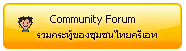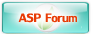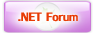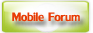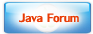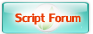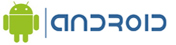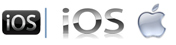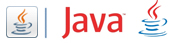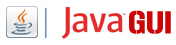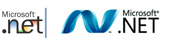Free Tutorial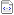สอน Google Maps Apiสอน Windows Serviceสอน Entity Frameworkสอน Androidสอน Java เขียน JavaJava GUI Swingสอน JSP (Web App)iOS (iPhone,iPad)Windows PhoneWindows AzureWindows StoreLaravel FrameworkYii PHP Frameworkสอน jQueryสอน jQuery กับ Ajaxสอน PHP OOP (Vdo)Ajax Tutorials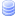SQL Tutorialsสอน SQL (Part 2)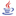JavaScript TutorialJavascript TipsVBScript TutorialVBScript ValidationMicrosoft Access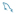MySQL Tutorials-- Stored ProcedureMariaDB Database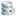SQL Server TutorialSQL Server 2005SQL Server 2008SQL Server 2012-- Stored ProcedureOracle Database-- Stored ProcedureSVN (Subversion)แนวทางการทำ SEOปรับแต่งเว็บให้โหลดเร็วลองค้นหาสิ่งที่ต้องการLoad balance : Server 02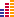© www.ThaiCreate.Com. 2003-2023 All Rights Reserved. ไทยครีเอทบริการ จัดทำดูแลแก้ไข Web Application ทุกรูปแบบ (PHP, .Net Application, VB.Net, C#) [Conditions Privacy Statement] ติดต่อโฆษณา 081-987-6107 อัตราราคา คลิกที่นี่